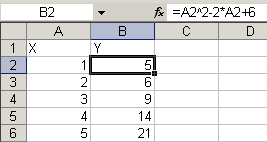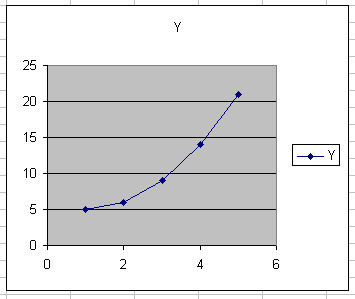# Charting a mathematical equation using Excel and defined names

## Introduction

When doing mathematics, wouldn't it be nice if we could type an equation into a cell in Excel and immediately see the resulting graph?

Suppose we have an equation like:

y=x2-2.x+6

Normally, we would create a column with x values and in the adjacent column "translate" this formula into a cell formula:

=A2^2-2*A2+6

and copy this formula down to match the number of x values in column A:Subsequently, we would base a chart on this set of data:This method lacks flexibility. It would be much more convenient, if we could:

• Enter the (any!) formula into a single cell
• Enter the lower and upper limit of the x-range into two other cells
• Enter the number of values to use for the chart in yet another cell:Yet another constraint we're going to place on this task:

Apart from the cells shown in the figure above, no cells are to be used, just defined names with formulas.